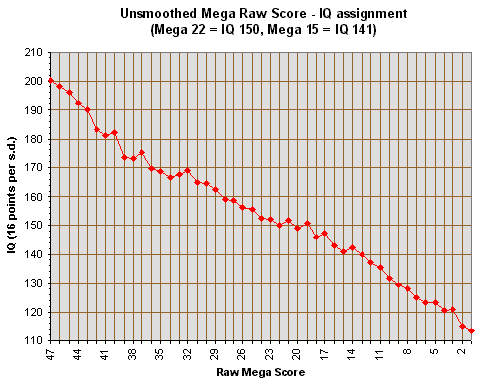Playing with the Calibration

Darryl Miyaguchi

Introduction
Playing with the Calibration

Microsoft Excel 3.0 file [no longer available] containing ability estimate (Rasch scores) for overall Mega Test raw scores from 1 to 47.

Probable IQs were calculated by Grady Towers on the assumption that a raw Mega Test score of 22 equals an IQ of exactly 150, and that a raw Mega Test score of 15 equals an IQ of exactly 141. An unsmoothed plot of these IQs using Grady's ability estimates are shown below:Since any two IQ values can be chosen to calibrate the Rasch scores, these assignments are not set in stone. For instance, I chose two IQ values that are contained within the linear portion of the curve produced in the sixth norming of the Mega Test. The unsmoothed plot is shown below. Interestingly, the one-in-a million level (IQ 176) occurs at a Mega Test raw score of 43, just as it does in Ron Hoeflin's sixth norming.